×
Get Full Access to UNL - Chem 109 - Class Notes - Week 9
Get Full Access to UNL - Chem 109 - Class Notes - Week 9

×

UNL / Chemistry / CHEM 109 / How do electron configurations fill orbitals?

# How do electron configurations fill orbitals? Description

Chapter 8: Periodic Properties of the Elements

## How do electron configurations fill orbitals?∙ Section 8.3

o Mendeleev’s periodic table is based on periodic law which  is when elements are arranged in increasing mass certain  properties reoccur periodically

o Periodic Property: One that is predictable based on an  element’s position within the periodic table

First

Electron

N=1

L=0

Ml=0

Ms=+1/2

Second

Electron

N=1

L=0

Ml=0

Ms=(-1/2)

## What are the three ways to indicate electron configuration?We also discuss several other topics like What is autocrine cellular signalling?

o Elect ron

1s2

Orbital

Number of electrons in orbital

Configuration: Shoes the particular orbitals that electrons  occupy for that atom

 Helium  2 electrons

∙ **Minimum Energy Principle (MEP): Always  obtain the lowest energy

∙ The above configuration is the ground state  configuration of He, the lowest energy

configuration for He

o Ground State: Lowest Energy

Configuration

o Excited State Highest Energy

Configuration

∙ Another way to show the electron configuration for elements is using orbital diagrams:

## What is the shielding effect of electrons?Symbolizes the electrons as an arrow and the  orbital as a box

o Orbital diagram for He:

**Direction of the

arrow corresponds

to the electron spin

∙ (+1/2)

o Coulomb’s Law 1s

corresponds  to up arrow

∙ (-1/2)

corresponds

to down Q’s are chargesDon't forget about the age old question of What are relatively liquid assets?

E=1 4πε

Q1Q2 R

R is distance

Es>Ep>Ed> Efo Orbital Stability

 Shielding: Inner electrons shield the nucleus from  the outer electrons

∙ ZEFF: Effective Nuclear Charge (ZEFF= Protons –

Inner e-)

o Aufbau Principle: “Build-up” Principle

Experiences net charge of about 1+

Shielding

e--

3+

Nucleus

e--

e--

Penetration Don't forget about the age old question of What is the meaning of the ovary?

Experiences full 3+ charge

e--

e-- If you want to learn more check out What is the temperance movement?
Don't forget about the age old question of Can you integrate a power series?

3+ Nucleuse--

 1st electron: lowest energy  2nd electron: next lowest energy  3rd electron: next lowest energy

 and so on. . .

 The point of the principle is to fill the lowest energy

orbital first:

∙ Example: Lithium If you want to learn more check out How many electrons are transferred in redox reaction?

Li  1s22s1

∙ The lowest energy orbital (1s) is filled up first

before the rest

o Hund’s Rule: Degenerate orbitals (same energy) fill with

electrons of same spin first (Don’t pair up electrons until

you have to!)

 Example: What are the 4 quantum numbers for the

highest energy electron in a ground state Oxygen

atom?

∙ **Oxygen has 8 electrons : 1s22s22p4

Box notation for a ground state oxygen atom

1s 2s 2p

o Objective: Be able to translate to box notation

 Summarizing Orbital Filling

∙ Electrons occupy orbitals so as to minimize the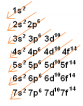energy of the atom; therefore, lower energy

orbitals fill before higher energy orbitals

(Diagonal Diagram )

∙ Orbitals can hold no more than two electrons

each. When two electrons occupy the same

orbital, their spins are opposite. This is another

way of expressing the Pauli Exclusion principle

(no two electrons in one atom can have the

same four quantum numbers)

∙ When orbitals of identical energy are available,

electrons first occupy these orbitals singly with

parallel spins rather than in pairs. Once the

orbitals of equal energy are half full, the

electrons start to pair ( Hund’s Rule)

∙ Section 8.4

o Valence Electrons: Electrons involved in bonding. Held

most loosely to the nucleus which is why they are easier to

share

 Outer electrons AND UNFILLED d electrons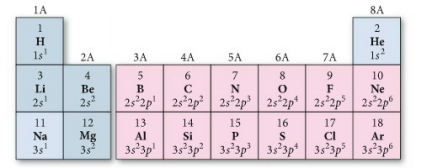 Trick: The group number of main group elements is  the number of valence electrons the element has.

∙ Example: Nitrogen (N)  Group 5A  5 Valence

electrons

∙ Example: Fluorine (F)  Group 7A  7 Valence

electrons

o Core Electrons: All non-valence electrons

o Inner Electrons: Lower n-value

o Outer Electrons: Highest n-value

o Example: Titanium (name valence, core, inner, and outer)

1s22s22p63s23p64s2

3d2

 Inner  20 electrons (lowest n-value(s))

 Outer  2 electrons ( highest n-value)

 Valence: Highest n-value + any unfilled d orbital

electrons

∙ 4s2 + 3d2  2+2=4 valence electrons

 Core: All the rest  22-4=18 core electrons

o Summarizing Periodic Table Organization

 The periodic table is divisible into four blocks

corresponding to the filling of the four quantum

sublevels (s, p, d, f)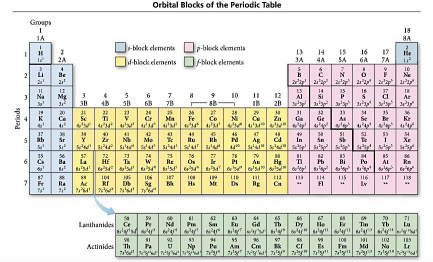 The group number of main-group element is equal to  the number of valence electrons for that element

 The row number of main-group element is equal to  the highest principle quantum number of that

element

o Objective: Determine electron configuration, valence  electrons, and core electrons based on position in periodic  table

o Nobel Gas Notation i.e. Core Notation

 [Ar] 4s2  the core notation for Calcium (Ca)

 Exceptions:

∙ Cr  [Ar] 4s23d4 BUT actual configuration is [Ar]

4s13d5 because a half full and full orbital is

more stable than something less or more

∙ Mo

∙ Cu

∙ Ag

∙ Section 8.5

o Na  1s22s22p63s1  unstable because subshell is not full,  causing sodium to want to loose electrons

 Na+  1s22s22p6

∙ Now by loosing an electron, all subshells are

full, making it stable. There are not more

protons ( since # of protons never change)

than electrons resulting in a (+) charge

∙ Section 8.6

o Key ideas . . .

 N-value

 ZEFF

s

u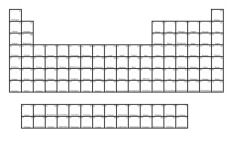i

d

a

R

c

i

m

o

t

A

g

n

i

s

a

e

r

c

n

I

o Van der Waals radius/ non-bonding atomic radius: radius of  an atom when it is not bonded to another atom

 Nonmetals: ½ the distance between 2 of the atoms

bonded together

 Metals: ½ the distance between 2 of the atoms next

to each other in a crystal of the metal

∙ Example: Distance between Br atoms is Br2 is

228 pm

Br Br

228pm

o Core electrons efficiently shield electrons in the outermost  principle energy level from nuclear charge, but outermost  electrons do not efficiently shield one another from nuclear charge

o Summarizing atomic radii for main-group elements

 As we move down a column in the periodic table, the

principal quantum number (n) of the electrons in the

outermost principal energy level increases, resulting

in larger orbitals and therefore larger atomic radii

 As we move to the right across a row in the periodic

table, the effective nuclear charge experienced by

the electrons in the outermost principal energy level

increases, resulting in a stronger attraction between

the outermost electrons and the nucleus and smaller

∙ Section 8.7

o Objective: Write electron configurations for any ion o Negative ions follow the rules

 Cl  [Ne] 3s23p5

 Cl-  [Ne] 3s23p6

∙ (-) adds to exponent (electrons)/ subshell; (+)  subtracts exponents (electrons)/ subshells

o Positive ions  outermost first

 Geranium  usually is a Ge2+ and Ge4+

 Just the element: [Ar] 4s23d104p2

o Transition Metals

 Remove the electron in the highest n-value orbitals  first, even if it does not correspond to the reverse  order of filling

o V: [Ar] 4s23d3

o V2+: [Ar] 4s03d3

o Magnetic Properties

 Objective: Determine if atom/ion has magnetic  properties

 Spin state of the electron produces a magnetic field ∙ Any unpaired electron will produce a magnetic  field

∙ Diamagnetic: Does not have a magnetic field  (all electrons are paired)

∙ Paramagnetic: Does have a magnetic field (at  least 1 unpaired electron)

o Example: Silver (Ag)  47 electrons

 Paramagnetic

 [Kr] 5s14d10

o **Just because there are an even number

of electrons does not mean it is

diamagnetic

∙ Objective: Rank ions by size and explain the

1s22s22p6

trend

1s22s22p63s1

ZEFF= (11 protons – 2

∙ Cation versus Atom (that cation is related too)

inner electrons) =

o Na+ v.s. Na

ZEFF= (11 protons –  10 inner electrons)

+9 Therefore, cation is smaller than atom

= +1

because of the difference in the effective

nuclear charge

∙ Cation. . . IF isoelectric

o Isoelectric: exact same electron

configuration BUT NOT the same number

of electrons

o Mg2+  1s22s22p6  Same electron

configuration as Neon

 The greater the charge the smaller

the ion

o Objective: Be able to compare a cation to

another cation and a cation to its parent

atom

∙    Anions. . . adding electrons

o F  1s22s22p5

o F-  1s22s22p6

 The anion has more electron

electron repulsion

o Compare: anion to parent atom  anion is

much larger!

o If isoelectric. . .

 Greater negative charge

o Ionization Energy (endothermic + ∆ H)

 Energy required to remove one electron from a gas

phase atom or ion

∙    IE1= Mg(g)  Mg+(g) + 1e--; IE2= Mg+(g) Mg2+

(g) + 1e—

∙    Determine ionization energy (IE) (each removal

of 1 electron is a different ionization energy)

 Objective: Rank atoms/ions by ionization energy

Ionization energy increases left to right (gets harder to pull electrons out because the effZEFF Ionization energy increaseEffective nuclear charge is huge when trying to  take second IE because  sodium wants to be a  +1 cation not a +2

∙ IE1 Na  1s22s22p63s1 (+1 ZEFF)  Na+ 

1s22s22p6 (+9)

 Summarize IE for Main-Group elements: ∙ Ionization energy generally decreases as we  move down a column (or family) in the periodic table because electrons in the outermost

principal level are increasingly farther away

from the positively charged nucleus and are

therefore held less tightly

∙ Ionization energy generally increases as we

move to the right across a row (or period) in

the periodic table because electrons in the

outermost principal energy level generally

experience a greater effective nuclear charge

∙ Section 8.8

o Electron Affinity: Energy change associated with adding an  electron to a gas phase atom/ion (exothermic reaction:

releases heat - ∆ H)

 O(g) + 1e--  O(g)— EA1

 O-- + 1e--  O2-(g) EA2

∙    EA1  closer to electron configuration; larger

negative value

o Summarizing Electron Affinity:

Decrease:

Size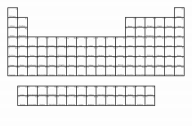Metallic Character

Increase:

Ionization Energy

Negative Electron Affinity

 Most groups (columns) of the periodic table do not

exhibit any definite trend in electron affinity. Among

the group 1A metals, however, electron affinity

become more positive as we move down the column

(adding an electron becomes less exothermic)

 Electron affinity generally becomes more negative

(adding an electron becomes more exothermic) as

we move to the right across a period (row) in the

periodic table

o Trends in Metallic Character

 Metallic Character: Behave like a metal (decreases

up and across periodic table)

Chapter 9: Chemical Bonding I – Lewis Model

∙ Section 9.1

o Lewis Model: Valence electrons represented as dots

produces Lewis Electron Dot Structures (Simplest electron  model)

o Chemical Bonding: MEP (minimum energy principal);

minimize the energy

 Chemical bonds form because they lower the

potential energy between the charged particles that

compose atoms

Types of Atoms

Type of Bond

Characteristic of Bond

Metal + Nonmetal

Ionic

Electron transferred

Nonmetal +

Nonmetal

Covalent

Electron Shared

minimize Metal + Metal

Metallic

energy

Proton + Proton Repulsion Electron Pooled

Electron + Electron Repulsion

maximize energy Electron + Proton Attraction

 The closer to the nucleus the more stable

∙ Section 9.2

o Objective: Describe each type of bonding

o Ionic: Electron transfer (Metal + Nonmetal)

 Example: Sodium Chloride

Does not

conduct

electricit

y

Conducts

electricity

(Delocaliz

ed

electrons)

Na+[ Cl ]-

o Covalent: Electron sharing (Nonmetal + Nonmetal)  Example: H2O

 Metallic: Electron sharing (Just metals are present i.e. Metal + Metal or Metal by itself)

o “Electron Sea Model”

 All of the atoms in a metal lattice pool their valence  electrons

 Pooled electrons no longer localized on a single atom but delocalized over the entire metal

∙ Section 9.3

o Objective: Draw Lewis Symbol for any main group element  o Examples:

Li Be B C N O F Ne s1 s2 s2p1 s2p2 s2p3 s2p4 s2p5 s2p6  o Octet Rule: Everything wants to have the noble gas  configuration usually stable arrangements have 8 electrons  o Duet: Hydrogen and Helium are stable with 2 electrons ∙    Section 9.4

o Objective: Represent ionic compound with Lewis symbols

NaCl Na + Cl Na Cl

Wrong Structure!

Sodium clears out 3rd orbital so it can have a noble gas configuration Chlorine takes sodium’s electron to get an octet

Na+ + Cl- Na+[ Cl ]-

Right Structure!

o Lattice Energy: Energy change when gas phase ions form a solid; Energy associated with the formation of a crystalline  lattice of alternating cations and anions from the gaseous  ions

 Example: NaCl

∙    Na+(g) + Cl—(g)  NaCl(s) ∙    But where does this energy come from??

Releases energy in  the process

∙    The transfer of an electron from sodium to  chlorine, by itself, actually absorbs energy  Objective: Use Born-Haber cycle to calculate lattice  energy (an application of Hess’s Law)

∙    Steps:

o 2. Get everything into gas phase

o 3. Get all to gas phase atoms  because  with ionic substances, ionization energies

are atom by atom

o Get all to gas phase ions

o Calculate Lattice Energy

 All components including lattice

energy add up to heat of the

reaction

 Ex: Construct Born-Haber Cycle and find Δ HLat KBr

K(s) + ½ Br2(s) KBr(s)

Hsublimation

Hvaporization x ½

K:

Hsub = 77.08 kJ/mol  IE = 418.6 kJ/mol

Br:

BDE = 193 kJ/mol

Solve for Lattice energy

K(g)

½ Br2(g)

EA = -324.6 kJ/mol Hvap = 29.96 kJ/mol

IE1BDE x ½

KBr:

Hf= -393.8 kJ/mol

K+(g)

Br(g)

EA1

Hf= Hsub + IE + ½ Hvap + ½ BDE + EA + HLat

-393.8 = 77.08 + 418.6 + ½ (29.96) + ½ (193) + (-324.6) + HLat

HLat= (-677) kJ/mol

Br—(g)

 Objective: Rank compounds by their lattice energies ∙    Example: Arrange these ionic compounds in

order of increasing magnitude of lattice energy:

CaO, KBr, KCl, SrO

o KBr and KCl should have lattice energies

smaller than SrO and CaO because of

their charges. SrO and CaO have +2 and

-2 and KBr and KCl have +1 and -1

charges

o Br is bigger than Cl because of atomic

size

o CaO and SrO should have larger lattice

energies because their charges are larger

than the others

o SrO has a larger ionic radius which

equals a lower magnitude

o KBr< KCl< SrO< CaO

∙    Highest Lattice Energy  Biggest negative

number

∙    Lowest Lattice Energy  Smallest negative

number

∙    Size is smaller. . .

**Charges always trump  size. SO if it comes to  equal charge in two  different compounds, size

o Lattice energy is larger negative value o NaF  Na= +1 and F= -1

o CaO  Ca = +2 and O= -2

 The charges change the Q values

in Coloumb’s equation

 The larger the charge, the larger

the lattice energy overall

 Summarizing Lattice Energy Trends:

∙    Lattice energies become less exothermic (less

∙    Lattice energies become more exothermic

(more negative) with increasing magnitude of

ionic charge

∙    Section 9.5

o Lewis Structures: Representing valence electrons in  molecules and polyatomic ions

o Objective: Draw Lewis Structures

 Example: H2O

 Example: O2

H O H H O H

O O Single Bond

Lone pair/ nonbonding pair

 Example: N2

∙    Section 9.6

N N

IncreaseDouble Bond Triple Bond

Bond Strength:  Single: lowest  strength

Double

 Triple: Better  stability/ strongest

Bond Length:

o Electonegativity: Ability to hold onto/ attract shared electrons (“Greed factor”)

o Objective: Rank by electronegativity  Inversely related to atomic

size: larger the atom, less

ability it has to attract

electrons to itself in a

covalent bond

 Main-group element trends

 Single: Longest  Double

 Triple: Shortest

Noble gases are not  included because they  are stable

∙    Electronegativity generally increases across a  period in the periodic table

∙    Electronegativity generally decreases down a  column in the periodic table

∙    Fluorine is the most electronegative element ∙    Francium is the least electronegative element  (sometimes called electropositive)

o Bond Polarity: Partial charge across a bond due to unequal  sharing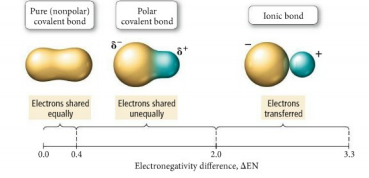 Classify Bond Polarity

∙    Pure covalent: equal sharing (Ex: Cl2)

Cl Cl

∙ Polar covalent: unequal sharing

H

Cl

∙ Ionic (nonmetal + metal)

o Electron almost completely transferred

Na+ Cl--

 Measure Dipole Moment: Anytime there is a  separations of positive and negative charge

∙    Section 9.7

o Rules for Drawing Structures

 1. Skeletal Structure

∙    H atoms are always terminal( i.e. at the ends)

∙    More electronegative atoms actually tend to go

to the outside of the molecule

∙    Less electronegative atoms will be more

central

 2. Total valence electrons

o Polyatomic ion: (-) ion  add an electron;

(+) ion  subtract an electron

 3. Distribute electron into molecule

∙    Fill octets ( or duets)

o Fill electrons in the order of outside to

inside

 4. Complete Octets (only if necessary)

 5. Minimize formal charges

∙    Section 9.8

o Resonance: Half the time the bond is single and the other  half its double or depending on the structure (Resonance  structure for Ozone also the best lewis structure for ozone)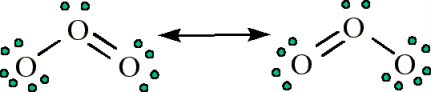o Hybrid resonance structure:

 Uses dotted lines to explain which bonds switcho Formal Charge

 Fictitous charge accounts for which element “claims”  with electron

Valence electrons as an element All lone electrons

 Formal Charge =

∙    Section 9.9

o Exceptions to the Octet rule:

- ( ½ Bonding electrons + )

 Odd # of valence electrons  free radicals

∙    Only write the best lewis structure that you

can!

Everything in this structure

has a formal charge of 0

therefore it is the best

lewis structure.

 Incomplete Octets

 Expanded Octets: No expanded octets for row 1 and  2 on periodic table

∙    Section 9.10

o Bond Energy: Energy required to break 1 mole of a bond Cl2 2Cl

VisuallyBreaking in ½

All fluorines have full  octets and the formal  charges are minimized  therefore this is the best  lewis structure even  though sulfur has 12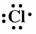o Objective: Estimate Δ Hf based on average bond energy

o **Key idea to remember: Not all bonds are the same, which

is why we are given AVERAGE bond energies

 Example: Estimate Δ Hf using bond energies for

CH4 + Cl2  CH3Cl + HCl

Bonds Broken Bonds Formed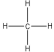+ +  A reaction is exothermic when weak bonds break and

strong bonds form

 A reaction is endothermic when strong bonds break

and weak bonds form

Bonds (Left Side):

4 C-H bonds (4)(414)

1 Cl-Cl bond (1)(243) = 1899

Bonds (Right Side):

3 C-H bonds (3)(414)

1 C-Cl bond (1)(339)

1 H-Cl bond (1)(431) = 2012

Broken – Formed = Δ Hf  1899 – 2012 = -

 Any bond formed is energy released into system  Any bond broken is energy absorbed

o Bond Length

 Objective: Rank bonds by length

∙     Bond Length:

o  Single: Longest

o  Double

o  Triple: Shortest

 How do you determine length differences in same  bonds?

Size decreases

F2

F-F

Cl2

Cl-Cl

Longe r

Page ExpiredIt looks like your free minutes have expired! Lucky for you we have all the content you need, just sign up here